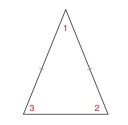Chapter 3.3, Problem 23E### Elementary Geometry for College St...

6th Edition
Daniel C. Alexander + 1 other
ISBN: 9781285195698

#### Solutions

Chapter
Section### Elementary Geometry for College St...

6th Edition
Daniel C. Alexander + 1 other
ISBN: 9781285195698
Textbook Problem
1 views

# In Exercises 23 to 28, use arithmetic or algebra, as needed, tofind the measures indicated. Note the use of dashes on equalsides of the given isosceles triangles. Find m ∠ 1 and m ∠ 2 if m ∠ 3 = 68 ° .To determine

To find:

The measure of m1 and m2.

Explanation

Given:

The given figure is,

Figure (1)

And,

m3=68°.

Approach:

(1) An isosceles triangle has two equal sides and two equal angles corresponding to the angles.

(2) The measure of sum of angles of a triangle is equal to 180°.

Calculation:

From figure (1),

m2=m3m2=68°

Equate the sum of angles of a triangle to 180°.

m1+m2+m3=180°(1)

Substitute 68°<

### Still sussing out bartleby?

Check out a sample textbook solution.

See a sample solution

#### The Solution to Your Study Problems

Bartleby provides explanations to thousands of textbook problems written by our experts, many with advanced degrees!

Get Started

#### Find the general indefinite integral. t(t2+3t+2)dt

Single Variable Calculus: Early Transcendentals, Volume I

#### In Exercises 914, evaluate the expression. 11. (3 4)2

Applied Calculus for the Managerial, Life, and Social Sciences: A Brief Approach

#### Multiply: (9)(+2)

Elementary Technical Mathematics

#### Which is the best graph of r = 1 − sin θ for 0 ≤ θ ≤ π?

Study Guide for Stewart's Single Variable Calculus: Early Transcendentals, 8th